# Pandas DataFrame Plot density graph

Pandas plot

Pandas.DataFrame.plot to generate density using data``````import pandas as pd
my_dict={
'MATH':[30,33,32,32,31,34,32]
}
df=pd.DataFrame(data=my_dict)
df.plot.density()``````

## density chart with options

There are several options we can add to above density diagram.

## title :

title='Student Mark' String used as Title of the graph.

## figsize :Size of the graph , it is a tuple saying width and height in inches, figsize=(6,3). Here width is 6 inches and height is 3 inches.
``df.plot.density(figsize=(6,3))``

## fontsize

fontsize=20 , we can set the font size used labels in x and y axis.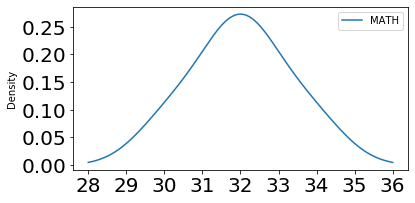``df.plot.density(fontsize=20)``

## color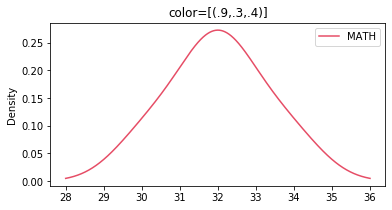We can use the option colors to give different colors to line . We can use one tuple to define the colours.

``df.plot.density(color=[(.9,.3,.4)])``

## style

This is a list or dict. We can specify how the style of the line.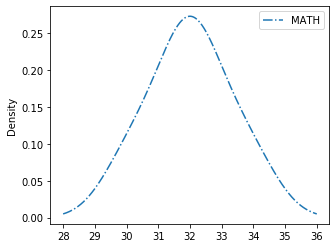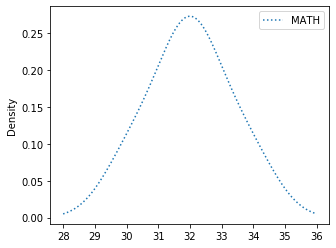``````my_style=['-.']
#my_style=[':']
df.plot.density(style=my_style)``````

## grid

We will show grid ( grid=True ) or not ( grid=False)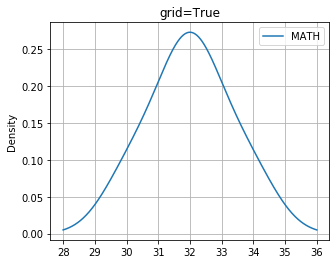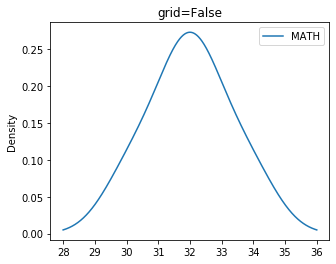## logx logy loglog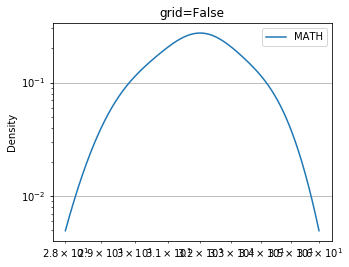We can specify log scaling or symlog scaling for x ( logx=True ) or y ( logy=True ) or for both x & y ( loglog=True)

``df.plot.density(loglog=True)``

## secondary_y

Whether to plot on secondary Y Axis ( secondary_y=True ) or not ( secondary_y=False )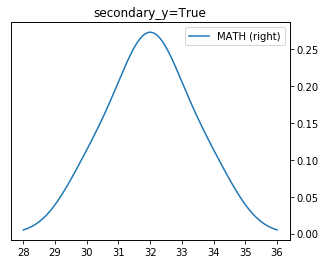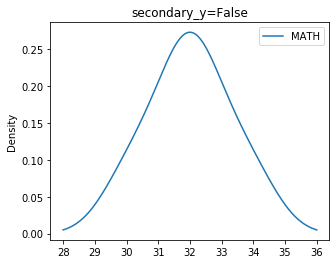``df.plot.density(secondary_y=True)``

## mark_right

Check the image above when secondary_y=True. There is a automatic marking in column lebels saying (right). We can manage this to show ( mark_right=True) or not ( mark_right=False)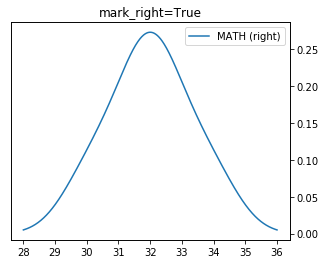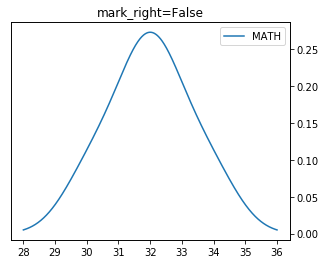``df.plot.density(mark_right=True,secondary_y=True)``

## rot

Rotation of ticks ( check the label at x axis )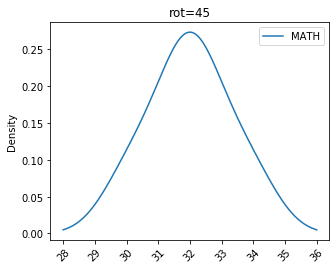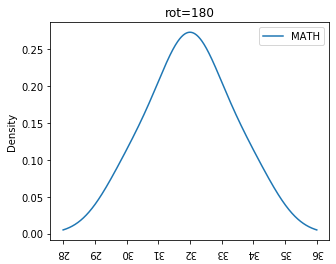``df.plot.density(title='rot=180',figsize=(5,4),rot=180)``

Subscribe to our YouTube Channel here

## Subscribe

* indicates required
Subscribe to plus2netplus2net.com